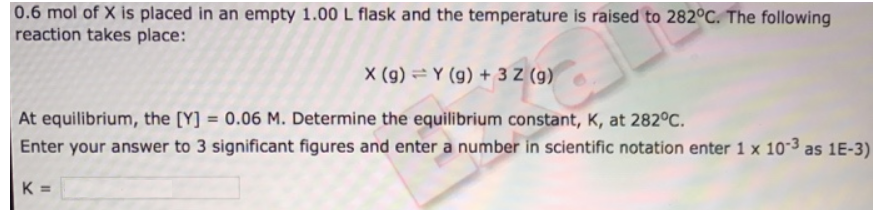# Problem: 0.6 mol of X is placed in an empty 1.00 L flask and the temperature is raised to 282°C. The following reaction takes place: X (g) ⇌ Y (g) + 3Z (g) At equilibrium, the [Y] = 0.06 M. Determine the equilibrium constant, K, at 282°C. Enter your answer to 3 significant figures and enter a number in scientific notation enter 1 x 10-3 as 1E-3).

###### FREE Expert Solution
98% (150 ratings)###### Problem Details

0.6 mol of X is placed in an empty 1.00 L flask and the temperature is raised to 282°C. The following reaction takes place:

X (g) ⇌ Y (g) + 3Z (g)

At equilibrium, the [Y] = 0.06 M. Determine the equilibrium constant, K, at 282°C. Enter your answer to 3 significant figures and enter a number in scientific notation enter 1 x 10-3 as 1E-3).